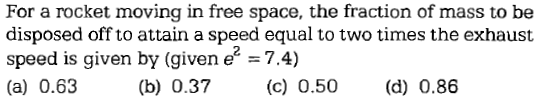NEET Questions SolvedConcept Videos :-

#29-Thrust-Force
30-Solved-Examples-14

Concept Questions :-

Variable mass system
Explanation is a part of a Paid Course. To view Explanation Please buy the course.

Difficulty Level:

If force on a rocket having exhaust velocity of 300 m/sec is 210 N, then rate of combustion of the fuel is

(1) 0.7 kg/s

(2) 1.4 kg/s

(3) 0.07 kg/s

(4) 10.7 kg/s

Concept Videos :-

#29-Thrust-Force
30-Solved-Examples-14

Concept Questions :-

Variable mass system
Explanation is a part of a Paid Course. To view Explanation Please buy the course.

Difficulty Level:

A 5000 kg rocket is set for vertical firing. The exhaust speed is 800 ms–1. To give an initial upward acceleration of 20 ms–2, the amount of gas ejected per second to supply the needed thrust will be (g = 10 ms–2)

(1) 127.5 kg s–1

(2) 187.5 kg s–1

(3) 185.5 kg s–1

(4) 137.5 kg s–1

Concept Videos :-

#29-Thrust-Force
30-Solved-Examples-14

Concept Questions :-

Variable mass system
Explanation is a part of a Paid Course. To view Explanation Please buy the course.

Difficulty Level:

A satellite in force-free space sweeps stationary interplanetary dust at a rate $dM/dt=\alpha v$ where M is the mass, v is the velocity of the satellite and $\alpha$ is a constant. What is the deacceleration of the satellite

(1) $-2\alpha {v}^{2}/M$

(2) $-\alpha {v}^{2}/M$

(3) $+\alpha {v}^{2}/M$

(4) $-\alpha {v}^{2}$

Concept Videos :-

#29-Thrust-Force
30-Solved-Examples-14

Concept Questions :-

Variable mass system
Explanation is a part of a Paid Course. To view Explanation Please buy the course.

Difficulty Level:

A rocket has a mass of 100 kg. 90% of this is fuel. It ejects fuel vapours at the rate of 1 kg/sec with a velocity of 500 m/sec relative to the rocket. It is supposed that the rocket is outside the gravitational field. The initial upthrust on the rocket when it just starts moving upwards is

(1) Zero

(2) 500 N

(3) 1000 N

(4) 2000 N

Concept Videos :-

#29-Thrust-Force
30-Solved-Examples-14

Concept Questions :-

Variable mass system
Explanation is a part of a Paid Course. To view Explanation Please buy the course.

Difficulty Level:

Rocket engines lift a rocket from the earth surface because hot gas with high velocity

(1) Push against the earth

(2) Push against the air

(3) React against the rocket and push it up

(4) Heat up the air which lifts the rocket

Concept Videos :-

#29-Thrust-Force
30-Solved-Examples-14

Concept Questions :-

Variable mass system
Explanation is a part of a Paid Course. To view Explanation Please buy the course.

Difficulty Level:

Sand is being dropped on a conveyor belt at the rate of M kg/s. The force necessary to keep the belt moving with a constant velocity of v m/s will be

(a)Mv newton

(b) 2 Mv newton

(c) $\frac{Mv}{2}$newton

(d) zero

Concept Videos :-

#29-Thrust-Force
30-Solved-Examples-14

Concept Questions :-

Variable mass system
Explanation is a part of a Paid Course. To view Explanation Please buy the course.

Difficulty Level: